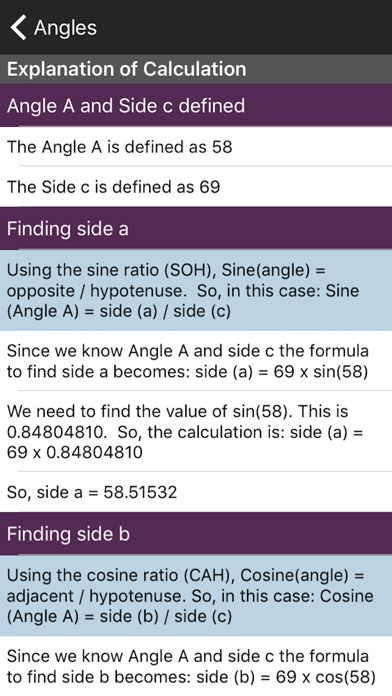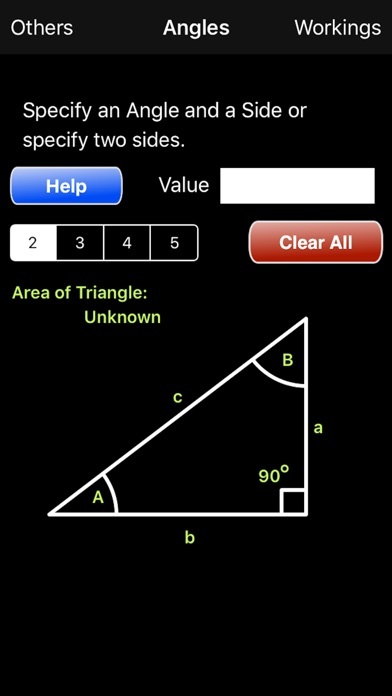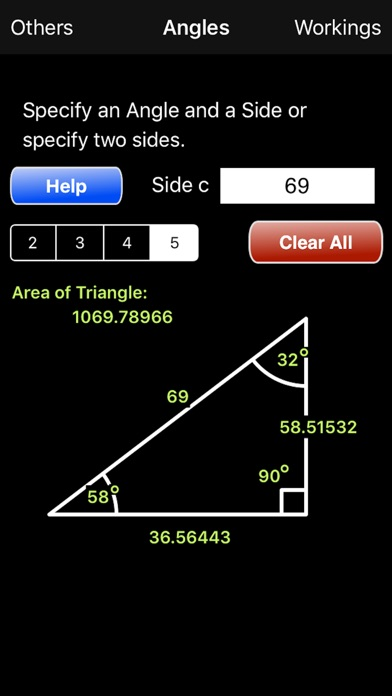Angles Calculator

iOS UniversalEducation

\$0.99

Do you need to learn how to determine the angles and lengths of a Right Angle Triangle? Need to clearly understand which of the sine, cosine and tangent ratios to use? Need to know how to determine the angle when you know two of the sides. If the answer is Yes to any of these questions then this App is for you. The Angles Calculator not only calculates the answers for you but it also tells you how it did it so you can understand how and when to apply the rules for right angle triangles.

New this version: Now the area of the triangle is calculated for you. An explanation of how this was done is also given.

The App is simple to use. Just enter an angle and a side or two sides and all the others will be calculated for you. To see how the answers were arrived at just select the 'Workings' button and a page is shown with a comprehensive set of steps to show how each was calculated.

To enter a value for an angle or a side just touch the diagram on or near the one you want to specify. The keypad pops up. Enter the value you want and select OK. Select the other value and do the same. When you press OK this time the App calculates the other values.

To start over select 'Clear All'. All values are reset. You can now specify two other values.

Note: The App cannot calculate the answers if you specify two angles and no sides. It is mathematically impossible. You have to specify an angle and a side or two sides.

•••